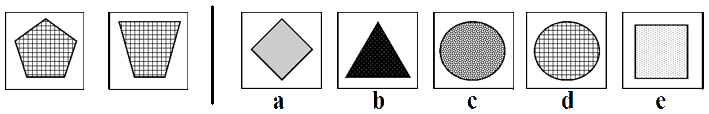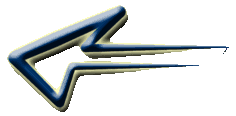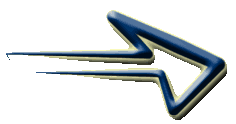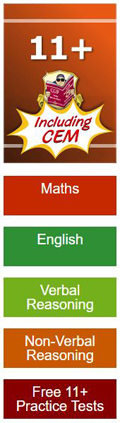Type 7 - 'Most Like'

With this type of question you are given two images to the left of a vertical line - These two images are alike in some way. To the right of the vertical line are five boxes labelled 'a' to 'e' containing symbols which are the possible answers.

You are required to determine which of the boxes to the right is most like the two images to the left.

Remember that the figures and diagrams consist of lines, shapes and shading/patterns. The shapes can shift in various ways. (rotate, reflect and transpose). The shapes can change in many ways. (increase/decrease, add and copy). They can consist of a movement, size or number sequence.Begin by looking at the two images on the left and try to see what they have in common.

Both images are filled by a cross hatching /netting pattern.

Now that you have discovered what the two images on the left have in common, it is just a case of comparing each of the images on the right to the images on the left and determining which of these has the same characteristics.

In this case it would be 'd' because the shape is also filled with the same pattern.

Practice working with Non Verbal Reasoning Type 7 questions with this
Non Verbal Reasoning Type 7 worksheetNon Verbal Type 7 worksheet